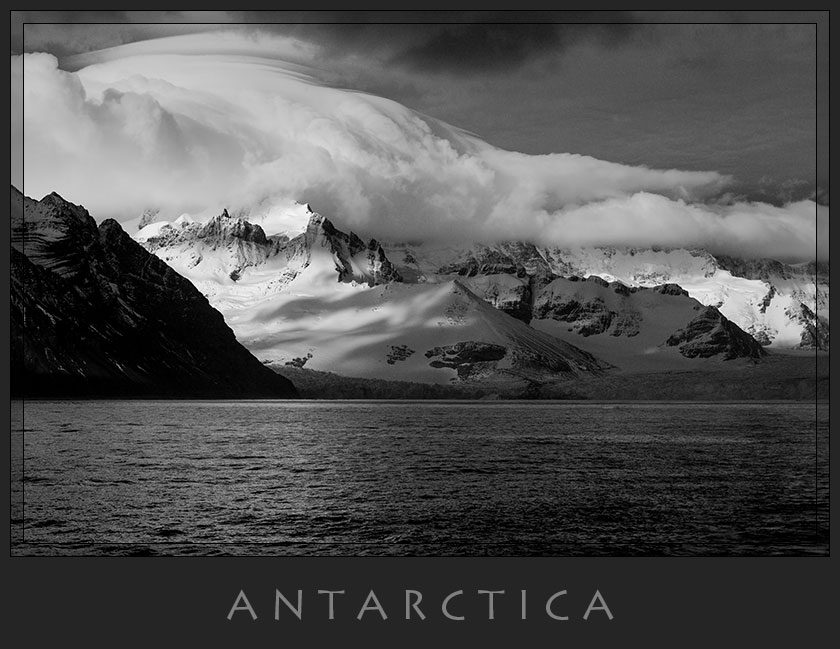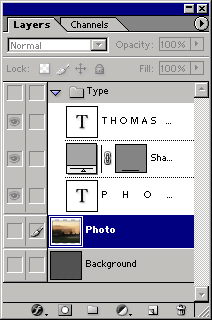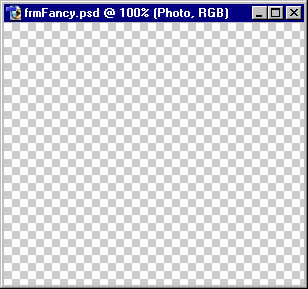# Poster## Description

A Poster frame may be downloaded as a Photoshop action. Before execution resize and sharpen the image — no need to flatten layers. The action creates the following layers:

 Document Background Photo Move Photo TitleAfter running the action choose the Move tool and adjust the Photo slightly upward with the Arrow keys to give a base to the picture and allow space for a title. A Lithos font was used for above image. Consider running the Black Border effect, after running the Poster action, for added separation from the background when posting on the internet.

There is a border that goes to the edge of the poster and within that border a translucent stripe surrounds the image. Thin lines are drawn on both sides of the translucent border. The following calculations that must be done before you run the action.

```PW = Poster Width
PH = Poster Height
IW = Image Width
IH = Image Height
BW = Border Width
TW = Translucent Width
LW = Line Width
TH = Title area Height

When running the action you will be prompted to enter the following information:
(2LW, 2LW) (TW) (LW) (PW, PH absolute)
```

Two sample calculations, using the image of Antarctica (4261 x 2841 px), are detailed for both a small and large poster.

## A Small Poster

```PW = 840
PH = unknown
IW = unknown
IH = unknown
BW = 10
TW = 12
LW = 1
TH = unknown

PW = IW + 2LW + 2BW

solving for IW and IH:
IW = PW - 2LW - 2BW = 840 - 2*1 - 2*10 = 818
IH = (2841/4261) * IW = (2841/4261) * 818 = 545

Let's see how much vertical space we are using:
IH + BW + 4LW = 535 + 10 + 4 = 549

Let's add 100 pixels for a title.
TH = 100
PH = 549 + TH =  549 + 100 = 649

With this information we can resize the image and determine the responses
required for the action:
image size = IW x IH = 818 x 545
(2LW, 2LW) (TW) (LW) (PW, PH absolute) = (2, 2) (12) (1) (840, 649)
```

## A Large Poster

The same image of Antarctica will now be used to create a 24" x 30" print. At 300 PPI a 1-pixel line hardly be visible so 3 pixels were used. A 1/2" border requires 1/2" x 300 PPI = 150 pixels. A 1/4" translucent stripe was achieved with 75 pixels. A poster height of 24" as assumed. Let's run through the calculations to see if there will be enough room for a title.

```PW = 9000 (30" x 300 PPI)
PH = 7200 (24" x 300 PPI)
IW = unknown
IH = unknown
BW = 150
TW = 75
LW = 3
TH = unknown

IW = PW - 2LW -2BW = 9000 - 2*3 - 2*150 = 8694
IH = (2841/4261) * IW = (2841/4261) * 8694 = 5796

Let's see how much vertical space we are using:
IH + BW + 4LW = 5796 + 150 + 4*3 = 5958

Deducting this from the poster height yields the amount of space for a title:
TH = PH - 5958 = 7200 - 5958 = 1242 px / 300 PPI = aprox 4" (got lucky!)

With this information we can resize the imageand determine the responses
required for the action:
image size = IW x IH = 8694 x 5796
(2LW, 2LW) (TW) (LW) (PW, PH absolute) = (6, 6) (75) (3) (9000, 7200)
```

After running the action the poster was exactly 24"x30" at 300 DPI.

## Construction

A selection for the translucent rim is made. After selection a dark layer is blended with a Darken blend and a stroke applied to yield the border. Finally a background layer is added.